# FreeX Retractable landing gear…Here the story, some days ago i buy a retractable DJI landing gear… i thought it will be fit for my freeX Drone.

With some small modification here and there the landing gear fit for my FreeX But i have some problem with it…

FreeX Skyview drone stock Radio transmitter have only 7CH, since i use Gimbal for my GoPro than i have use all the channel.

than how i can control my gear up and down?

The answer is, i use Arduino Pro Mini to generate extra channel from existing channel i have… and this is how to do it…

• Open your freeX upper fuselage, be careful with GPS cable attach on it.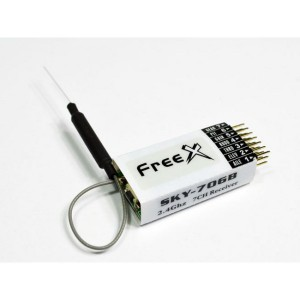• Parallel and soldering a socket pin to the Signal pin on CH6 (Signal pin is the first pin row from the top)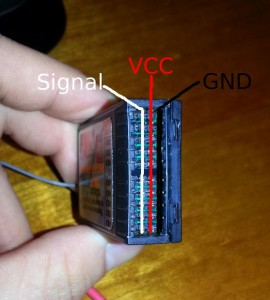• CH 6 is for headless / Altitude flying control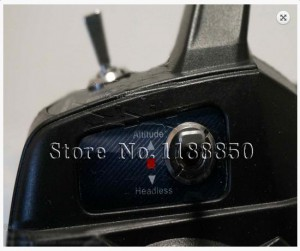• Using Socket cable, connect the GDN and Vcc from arduino pro mini to the GDN and Vcc of the receiver (second and third pin row ) for powering the arduino pro mini  board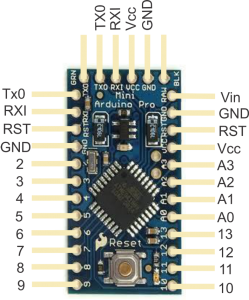• for input/output you can use any pin from 0 to 13, but in my case i use pin 5 for input and pin 8 for output
• Connect Arduino pin 5 (input) to the parallel pin you have make before, this pin function as listener from CH6.
• Look for gear controller (a black box include in the retractable gear package), there’s black and red cable for the power from the battery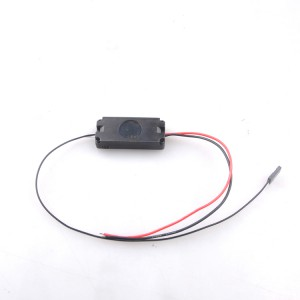• Still at the same box, Connect Arduino pin 8 (output) to the Signal input on the gear controller (White cable)
• connect 2 socket from the gear to the side pin of the box (be careful with + and – pin)
• Now the programming part… (klik + sign below)

Click to expand the code –> FreeXgear.ino

int ch5; // Here’s where we’ll keep pin to read our RC channel values
int loop1;int press1;int press2;int gear;
int timeout;
void setup() {
pinMode(13, OUTPUT);//set LED pin for visual indicator
pinMode(5, INPUT); // Set our input pins as such
pinMode(8, OUTPUT);// set pin for output signal
Serial.begin(9600); // Pour a bowl of Serial
loop1=0;press1=0;press2=0;gear=0;
timeout=30;
}

void loop() {
ch5 = pulseIn(5, HIGH, 25000); // Read the pulse width of pin 5
if (loop1<timeout) {
loop1=loop1+1;
} else {
press1=0;
press2=0;
}
if (ch5 < 1000) {
press1=press2+1;
loop1=0;
}else {
press2=press1;
}

// start ext ch w/ 2 loop
if (press2==2 && press1==2 && loop1==timeout) {
digitalWrite(13, LOW);
for (int i=0; i <= 10; i++){
digitalWrite(8, HIGH); // turn the LED on (HIGH is the voltage level)
delay(10); // wait for a second
digitalWrite(8, LOW); // turn the LED on (HIGH is the voltage level)
delay(10);
}

delay(10);
digitalWrite(13, HIGH);
for (int i=0; i <= 10; i++){
digitalWrite(8, HIGH); // turn the LED on (HIGH is the voltage level)
delay(1); // wait for a second
digitalWrite(8, LOW); // turn the LED on (HIGH is the voltage level)
delay(1);
}
press1=0;
press2=0;
}
if (loop1==timeout) {
press1=0;
press2=0;
}
// end ext ch w/ 2 loop
delay(10);
}

And this is the result

and my backpack modification…